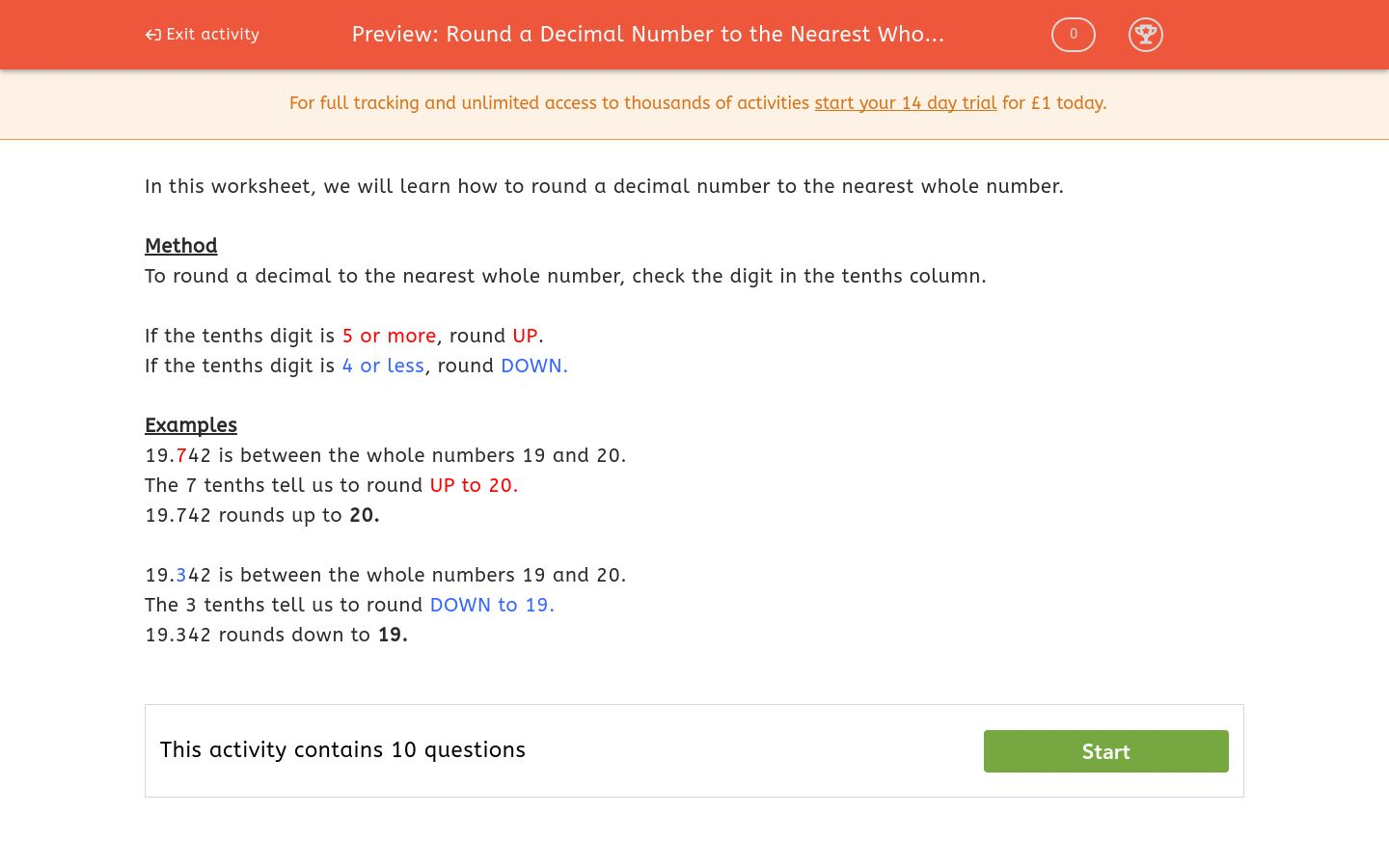# Round a Decimal Number to the Nearest Whole Number

In this worksheet, students round decimal numbers to the nearest whole number.Key stage:  KS 3

Curriculum topic:  Number

Curriculum subtopic:  Round/Approximate Numbers Appropriately

Difficulty level:### QUESTION 1 of 10

In this worksheet, we will learn how to round a decimal number to the nearest whole number.

Method

To round a decimal to the nearest whole number, check the digit in the tenths column.

If the tenths digit is 5 or more, round UP.

If the tenths digit is 4 or less, round DOWN.

Examples

19.742 is between the whole numbers 19 and 20.

The 7 tenths tell us to round UP to 20.

19.742 rounds up to 20.

19.342 is between the whole numbers 19 and 20.

The 3 tenths tell us to round DOWN to 19.

19.342 rounds down to 19.

Round the following number to the nearest whole number.

85.7192

Round the following number to the nearest whole number.

39.3561

Round the following number to the nearest whole number.

7.0114

Round the following number to the nearest whole number.

75.9869

Round the following number to the nearest whole number.

66.499

Round the following number to the nearest whole number.

58.4471

Round the following number to the nearest whole number.

41.9905

Round the following number to the nearest whole number.

559.4771

Round the following number to the nearest whole number.

200.905

Round the following number to the nearest whole number.

8008.6357

• Question 1

Round the following number to the nearest whole number.

85.7192

CORRECT ANSWER
86
EDDIE SAYS

The tenths column here is 7

85.7192

This means we have to round up.

• Question 2

Round the following number to the nearest whole number.

39.3561

CORRECT ANSWER
39
EDDIE SAYS

The tenths column here is 3

39.3561

This means we have to round down.

• Question 3

Round the following number to the nearest whole number.

7.0114

CORRECT ANSWER
7
EDDIE SAYS

The tenths column here is 0

7.0114

This means we have to round down.

• Question 4

Round the following number to the nearest whole number.

75.9869

CORRECT ANSWER
76
EDDIE SAYS

The tenths column here is 9

75.9869

This means we have to round up.

• Question 5

Round the following number to the nearest whole number.

66.499

CORRECT ANSWER
66
EDDIE SAYS

The tenths column here is 4

66.499

This means we have to round down.

• Question 6

Round the following number to the nearest whole number.

58.4471

CORRECT ANSWER
58
EDDIE SAYS

The tenths column here is 4

58.4471

This means we have to round down.

• Question 7

Round the following number to the nearest whole number.

41.9905

CORRECT ANSWER
42
EDDIE SAYS

The tenths column here is 9

41.9905

This means we have to round up.

• Question 8

Round the following number to the nearest whole number.

559.4771

CORRECT ANSWER
559
EDDIE SAYS

The tenths column here is 4

559.4771

This means we have to round down.

• Question 9

Round the following number to the nearest whole number.

200.905

CORRECT ANSWER
201
EDDIE SAYS

The tenths column here is 9

200.905

This means we have to round up.

• Question 10

Round the following number to the nearest whole number.

8008.6357

CORRECT ANSWER
8009
EDDIE SAYS

The tenths column here is 6

8008.6357

This means we have to round up.

---- OR ----

Sign up for a £1 trial so you can track and measure your child's progress on this activity.

### What is EdPlace?

We're your National Curriculum aligned online education content provider helping each child succeed in English, maths and science from year 1 to GCSE. With an EdPlace account you’ll be able to track and measure progress, helping each child achieve their best. We build confidence and attainment by personalising each child’s learning at a level that suits them.

Start your £1 trial